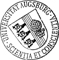Domain Decomposition and Model Reduction for the Numerical Solution of PDE Constrained Optimization Problems with Localized Optimization Variables

• We introduce a technique for the dimension reduction of a class of PDE constrained optimization problems governed by linear time dependent advection diffusion equations for which the optimization variables are related to spatially localized quantities. Our approach uses domain decomposition applied to the optimality system to isolate the subsystem that explicitly depends on the optimization variables from the remaining linear optimality subsystem. We apply balanced truncation model reduction to the linear optimality subsystem. The resulting coupled reduced optimality system can be interpreted as the optimality system of a reduced optimization problem. We derive estimates for the error between the solution of the original optimization problem and the solution of the reduced problem. The approach is demonstrated numerically on an optimal control problem and on a shape optimization problem.

• Dokument_1.pdfAuthor: Harbir AntilGND, Matthias Heinkenschloss, Ronald H. W. HoppeGND, Danny C. Sorensen urn:nbn:de:bvb:384-opus4-10652 https://opus.bibliothek.uni-augsburg.de/opus4/1253 Preprints des Instituts für Mathematik der Universität Augsburg (2009-11) Preprint English Universität Augsburg University of Houston, Rice University 2009/04/30 optimal control; shape optimization; domain decomposition; balanced truncation model reduction Optimale Kontrolle; Mathematisches Modell; Ordnungsreduktion; Partielle Differentialgleichung; Gebietszerlegungsmethode Mathematisch-Naturwissenschaftlich-Technische Fakultät Mathematisch-Naturwissenschaftlich-Technische Fakultät / Institut für Mathematik Mathematisch-Naturwissenschaftlich-Technische Fakultät / Institut für Mathematik / Lehrstuhl für Numerische Mathematik 5 Naturwissenschaften und Mathematik / 51 Mathematik / 510 MathematikDeutsches Urheberrecht mit Print on Demand